# Texas Go Math Kindergarten Unit 2 Assessment Answer Key

Refer to our Texas Go Math Kindergarten Answer Key Pdf to score good marks in the exams. Test yourself by practicing the problems from Texas Go Math Kindergarten Unit 2 Assessment Answer Key.

## Texas Go Math Kindergarten Unit 2 Assessment Answer Key

Vocabulary

Question 1.Explanation:
The sum of 3 orange cubes and 2 green cubes
3 + 2 = 5

Question 2.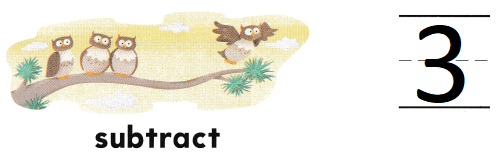Explanation:
There are 4 owls on a tree
1 flies away
4 – 1 = 3
so, owl are left on the tree.

Concepts and Skills

Question 3.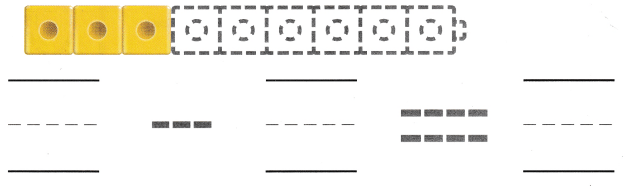Explanation:
Three cubes are yellow and the rest are blue.
9 – 3 = 6
so, 6 cubes are blue

Directions
1. Write the number of each color cubes. Write the number to show how many in all. TEKS K.3.A 2. Write the number to show how many are left. TEKS K.3.A. 3. Draw blue cubes to make a nine-cube train. Three cubes are yellow and the rest are blue. Write to complete the subtraction sentence. TEKS K.3.B

Question 4.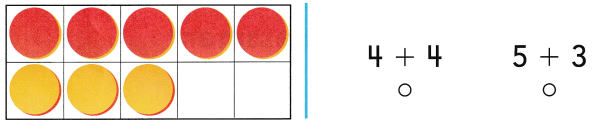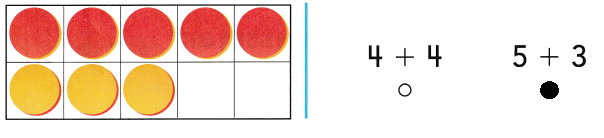Explanation:
In a ten frame there are 5 red counters
and 3 yellow counters
5 + 3 = 8 counters in a ten frame.

Question 5.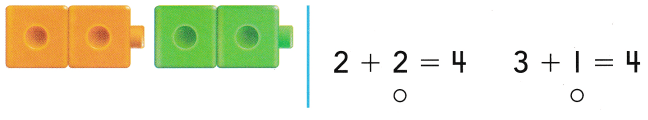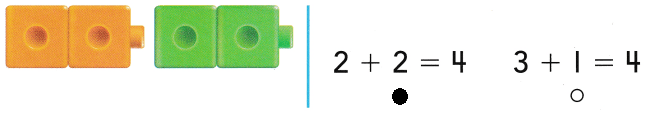Explanation:
The sum of 2 yellow cubes and the 2 green cube = 4
2 + 2 = 4

Question 6.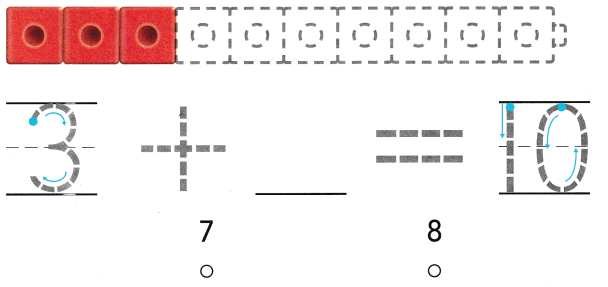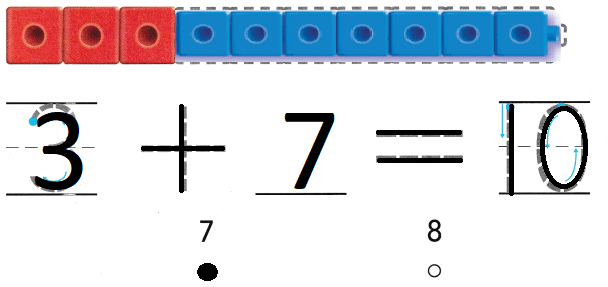Explanation:
There is a 10 cube train
3 cubes are red
7 cubes are blue
3 + 7 = 10

Directions
4. Mark under the addition that shows the sets put together. TEKS K.3.A. 5. Mark under the addition sentence that shows how many there are when the cubes are put together. 6. How many red cubes do you see? How many blue cubes do you need to odd to make 10? Use blue to color those cubes. Mark under the number that would complete the addition sentence. TEKS K.3.B

Texas Test Prep

Question 7.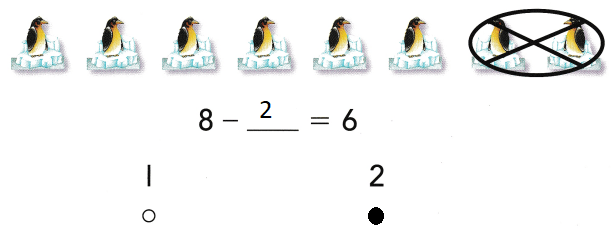Explanation:
There are 8 penguins in the group
2 penguins swam away
8 – 2 = 6
so, 6 penguins are left.

Question 8.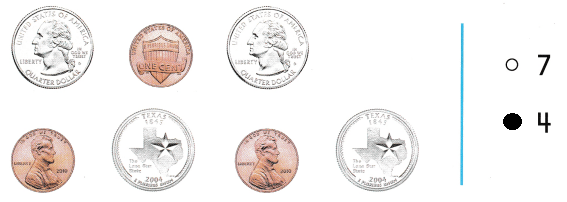Explanation:
There are 4 quarters in the group
so, marked the number 4

Question 9.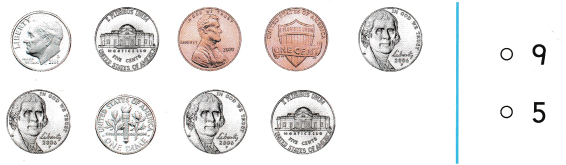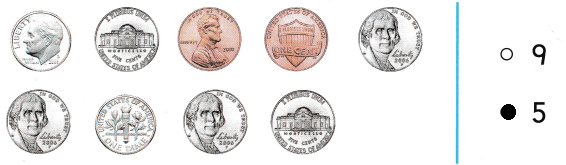Explanation:
There are 5 nickels in the above group
so, marked the number 5

Directions
7. Mark under the number that shows how many birds are being taken from the set. TEKS K.3.C 8. Mark beside the number that shows how many quarters are in this group. TEKS K.4 9. Mark beside the number that shows how many nickels are in this group. TEKS K.4Explanation:Explanation:
The sum of 1 and 2 is 3
1 + 2 = 3
The difference of 3 and 2 is 1
3 – 2 = 1